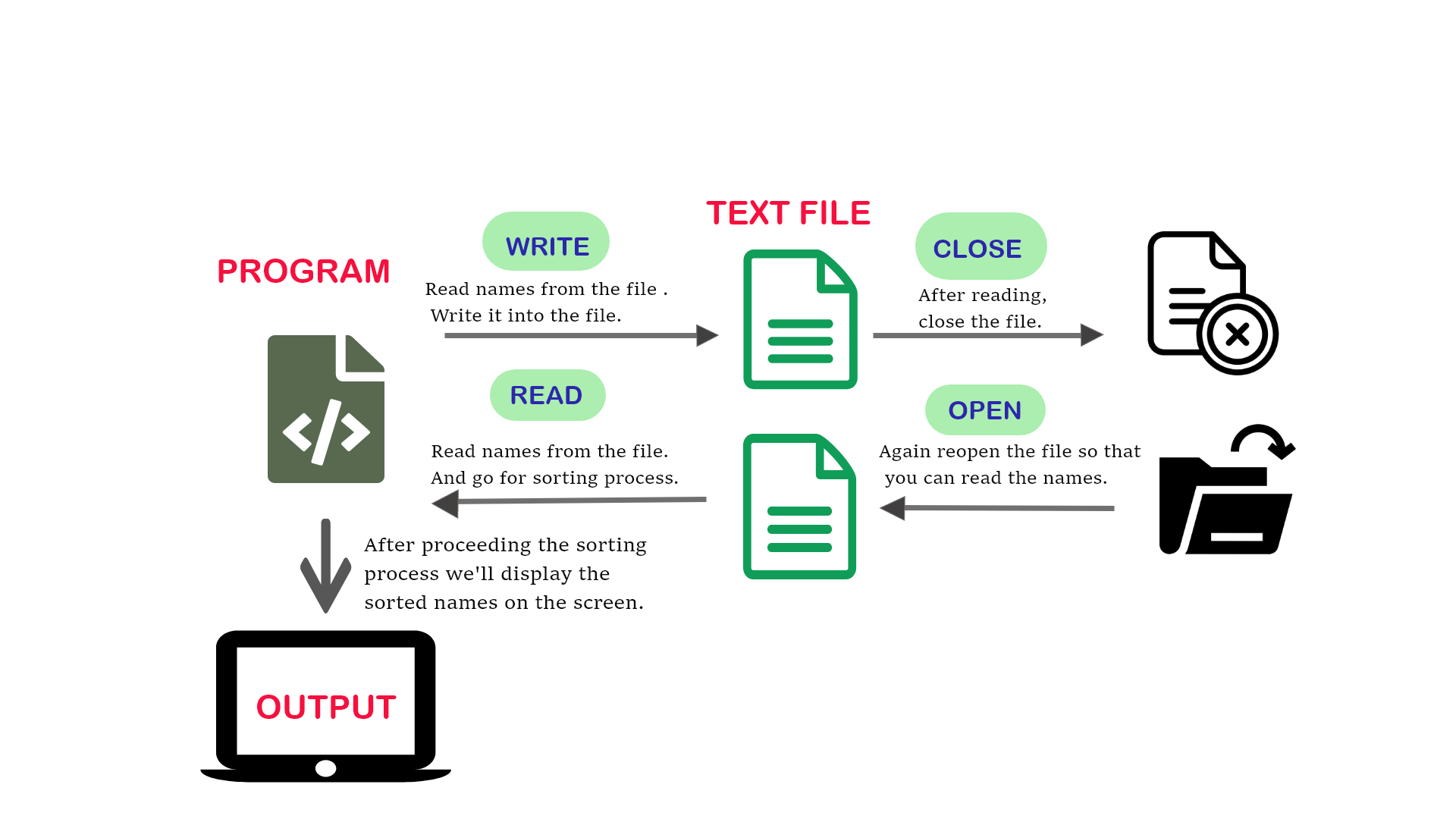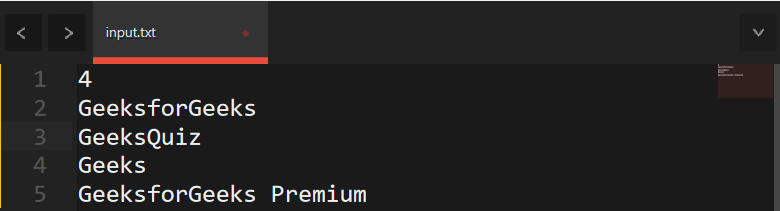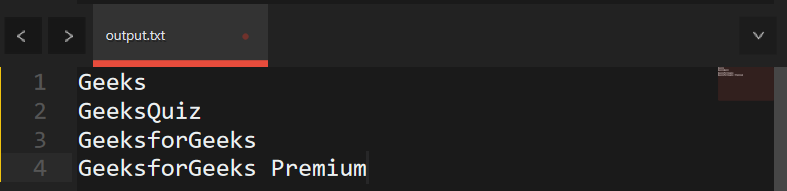Related Articles
Sorting strings from the text file
• Last Updated : 24 Nov, 2020

Given a text file “file.txt” that consists of strings, the task is to sort all the strings in alphabetical order in that text file.## Recommended: Please try your approach on {IDE} first, before moving on to the solution.

Approach: The idea is to use the concept of File Handling and a text file(say file.txt) that contains all the strings. Below are the steps:

Below is the implementation of the above approach:

## C++

 `// C++ program to sort given array``// of string stored in a file``#include ``#include ``#include ``#include ``using` `namespace` `std;`` ` `// Driver Code``int` `main()``{``    ``int` `N, i, j;`` ` `    ``// File pointer to open file``    ``FILE``* f;`` ` `    ``// fopen() for creating of a file``    ``f = ``fopen``(``"file.txt"``, ``"w"``);`` ` `    ``// Input number of strings``    ``// to be inserted in file``    ``cin >> n;`` ` `    ``vector<``int``> name(N);`` ` `    ``// Insert the strings into file``    ``for` `(i = 0; i < n; i++) {`` ` `        ``// Insert names in file``        ``cin >> name[i];`` ` `        ``// Writing into the file``        ``fprintf``(f, ``"%s"``, name[i]);``    ``}`` ` `    ``// Close the file``    ``fclose``(f);`` ` `    ``// Reopening in read mode``    ``f = ``fopen``(``"file.txt"``, ``"r"``);`` ` `    ``// Check does file exist or not``    ``if` `(f == NULL) {``        ``cout << ``"File doesn't exist!"``;``        ``return` `0;``    ``}`` ` `    ``// Read the file until it``    ``// encounters end of line``    ``while` `(!``feof``(f)) {``        ``fscanf``(f, ``"%s"``, name[i]);``        ``i++;``    ``}``    ``n = i - 1;`` ` `    ``// Sort the strings``    ``sort(name.begin(), name.end());`` ` `    ``// Insert the strings into file``    ``// after sorting``    ``for` `(i = 0; i < n; i++) {`` ` `        ``// Write into the file``        ``fprintf``(f, ``"%s"``, name[i]);``    ``}`` ` `    ``// Print the sorted names``    ``for` `(i = 0; i < n; i++) {``        ``cout << name[i] << ``'\n'``;``    ``}`` ` `    ``return` `0;``}`

Input File:Output File:Want to learn from the best curated videos and practice problems, check out the C++ Foundation Course for Basic to Advanced C++ and C++ STL Course for the language and STL.  To complete your preparation from learning a language to DS Algo and many more,  please refer Complete Interview Preparation Course.
My Personal Notes arrow_drop_up